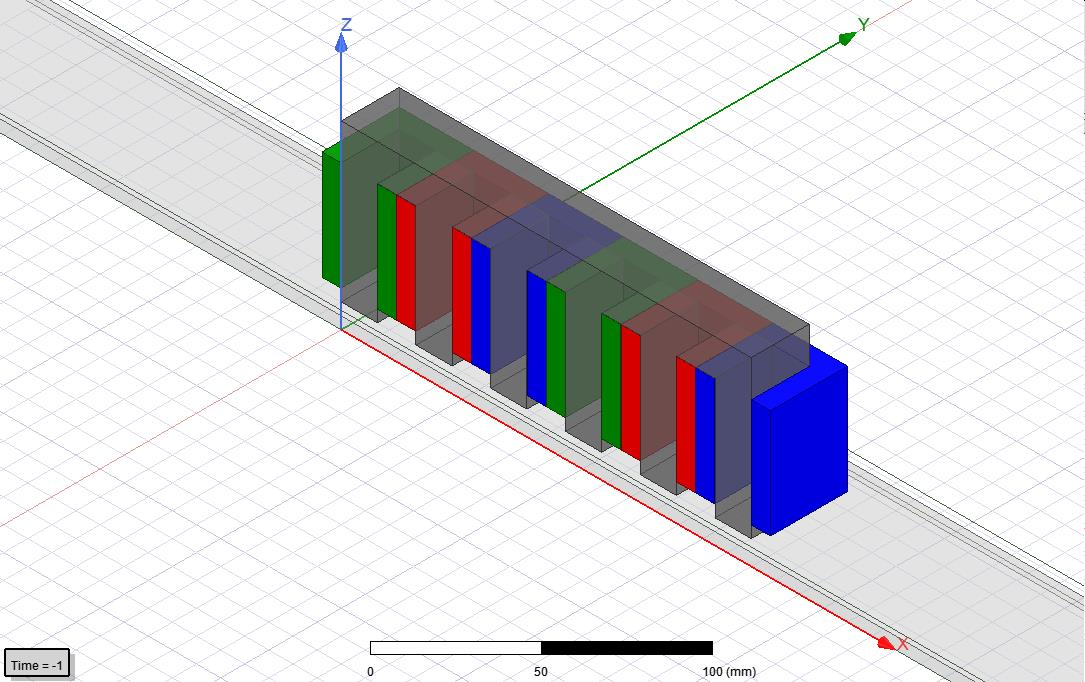# Voltage Simulation Linear Induction Motor

Hallo everyone

I am simulating a 1 pole pair double sided linear induction motor with concentrated slot windings as shown in the picture below. Simulating the forces works well and the next step is to estimate the induced voltages in the coils. The windings are modeled as stranded coil terminals with a time dependent current, which are grouped into windings.(I am working with two symmetry planes)

I already know that you can, and I also did enable the computation of the induction matrices. My goal is to simulate the induced voltages in the coil the estimate the voltage requirements for the inverter, especially at high speeds. Therefore, I have the following questions:

• Do I have to select apparent or incremental computation of the inductance matrix?
• Do I have to model the coils different, e.g. model single wires to get accurate and useful results?

Philip